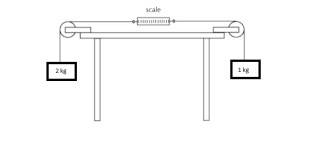# Use Newtonian Model to determine what the scale reads

xyphr

## Homework StatementThe strings attach to a scale which reads the force. What is the force that the scale will read?

## Homework Equations

F=ma according to Newton's Laws.

## The Attempt at a Solution

Thinking logically, I simply calculated the force of the left side:

F=ma => (2kg)(9.8m/ssq) = 19.6N

Then calculated the force of the right side:

F=ma => (1kg)(9.8m/ssq) = 9.8N

Then simply subtracted the two, leaving the final answer to be 9.8N. My teacher told me that this is not the right answer. I'm confused as to how else I could have done this problem. Thanks for the help!

Mentor
Thinking logically, I simply calculated the force of the left side:

F=ma => (2kg)(9.8m/ssq) = 19.6N
What you found here is not 'ma', but mg. That's the weight of the left mass. That's just one of the forces acting on that mass. What's the other?

Then calculated the force of the right side:

F=ma => (1kg)(9.8m/ssq) = 9.8N
Same issue here.

What you need to do is identify all the forces acting on each mass and then apply ƩF = ma to each mass separately. Then you can solve for the acceleration of the masses and the tension in the cable (which will equal the scale reading).